### Home > PCT > Chapter Ch9 > Lesson 9.3.3 > Problem9-134

9-134.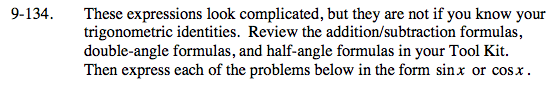Look in the index and/or glossary for these formulas.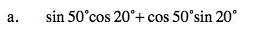Use the addition formula for sine.
sin(50° + 20°)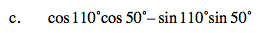Use the sum/difference formula for the cosine. Be careful of your sign.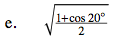Use the Half-Angle Formula for cosine.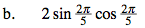Use the Double-Angle Formula for the sine.

$\text{sin}\left( 2\left( \frac{2\pi}{5} \right) \right)$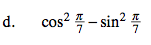Use one of the Double-Angle Formulas for the cosine.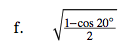Use the Half-Angle Formula for sine.